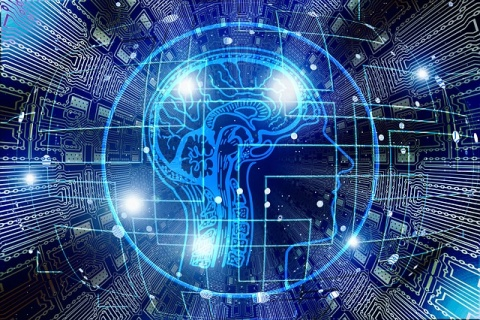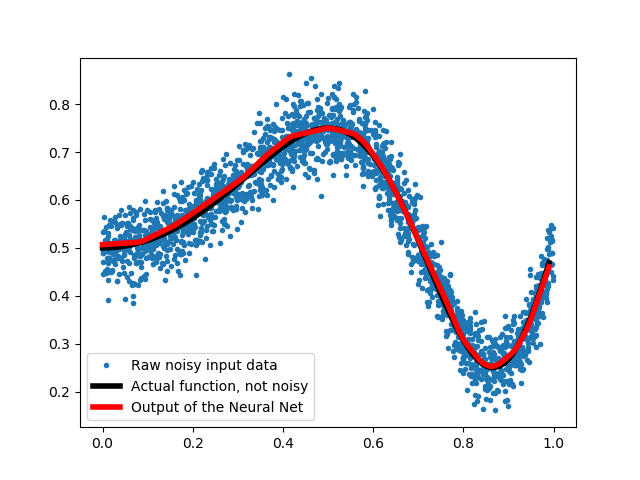Published in blog / machine-learning
Tags: Keras / Python / TensorFlowEver since the great success of reinforcement learning been shown  in the context of chess, I was very much interested in the possibilities of artificial intelligence and machine learning - the changes for society due to related technologies will be much larger than anything that has been seen by mankind , including the wheel, internet, and deep-fried pizza.

So, let's get a hand on it. Fortunately there exist a plethoria of high-level libraries with machine learning tutorials out there. Unfortunately, they are often still hard to grasp for a quick and dirty start. For example Google's TensorFlow is extremely comprehensive and might be the main player in the field for the upcoming years.

TensorFlow itself exhibits a somewhat steep learning curve making it less attractive for people who want to simply put up a neural net in a few minutes. Therefore, high-level neural network API's have been developed. They make it much more comfortable for the machine-learning-layman to deal with the subject. One of these API's is Keras. Keras is written in Python and aiming at user friendliness. Two good reasons to love it. Let's have a look how to get started.

## Prerequisites - Getting Keras to run on Windows

One big advantage of most Python libraries is that they simply work upon installation using Python's standard installer pip. Keras requires TensorFlow. However, TensorFlow may not be supported by the latest Python installation. Therefore, please follow these steps to get Python with Keras working on your compurter:

1. Visit the TensorFlow pip installation guide and write down the latest required Python version. As of today, that would be Python 3.6 (not the latest 3.7!)
3. Install Python on your hard drive. Check the "Add Python to the PATH Environmental Variable" mark if available - you should run python not only from the actual installation directory.
4. Install Keras and TensorFlow using pip:
``````    pip install tensorflow
pip install keras
``````

That's it! Using your favorite editor you are now officially dangerous to unleash the neural-net dragon!

## The actual code - A kind of Keras Neural Network "Hello World"

One of the ways to see a neural network is an interpolation function, for example for cases in which an analytic solution is not known. How would you strictly define a function that is able to characterize a cat or dog? Neural networks are exactly for such hard tasks and we will eventually come to this point.

For now, as a very basic example let us check how to interpolate a one-dimensional function from some noisy input data. Here is a quick walk through the code.

### Library Import

At first, all necessary libraries need to be imported. You can see that we need some of keras, some of numpy and some of matplotlib as well:

``````from keras.models import Sequential
from keras.layers import Dense
import numpy as np
import matplotlib.pyplot as plt
``````

### Teaching Input: A simple Function

As input I have chosen a rescaled and shifted sine function with squared argument. You may adjust the function to your likings. The rescaling and shifting has been done such that the neural net has input and output values between zero and one. This is adjusted to some of the so-called activation functions with output values in exactly that range.

Furthermore, using some Gaussian random noise the target function "Y" is established. Values for the argument "X" are chosen at random as well. For later plotting purposes, we also calculate non-noisy values "Yreal".

``````def inputfunct(x):
return 0.25*(np.sin(2*np.pi*x*x)+2.0)

np.random.seed(5)
X = np.random.sample()
Y = inputfunct(X) + 0.2*np.random.normal(0,0.2,len(X))

Xreal = np.arange(0.0, 1.0, 0.01)
Yreal = inputfunct(Xreal)
``````

### Establishing the Neural Network Model

And here comes the magic of Keras: establishing the neural network is extremely easy. Simply add some layers to the network with certain activation functions and let the model compile. For simplicity we have chosen an input layer with 8 neurons, followed by two hidden layers with 64 neurons each and one single-neuron output layer. Technically, this network is a deep neural network. Its implementation in Keras is really that simple:

``````### Model creation: adding layers and compilation
model = Sequential()

``````

### Train the Neural Network

After the neural network model is established, it needs to be trained. In this step the X values are used as input and compared to the target Y values. Then, the weights and biases of the neural network are adjusted in each learning iteration called epoch. Not all data is processed at the same time, only a certain number of batches.

``````nepoch = 128
nbatch = 16
model.fit(X, Y, epochs=nepoch, batch_size=nbatch)
``````

### Use the Neural Network for Value Predictions

After a number of epochs the model may be able to provide predictions. In our example we can simply feed the model with some arguments and see if the results are somewhat comparable to our target function. For completeness, the plot instructions are shown as well.

``````Ylearn = model.predict(Xreal)

### Make a nice graphic!
plt.plot(X,Y,'.', label='Raw noisy input data')
plt.plot(Xreal,Yreal, label='Actual function, not noisy', linewidth=4.0, c='black')
plt.plot(Xreal, Ylearn, label='Output of the Neural Net', linewidth=4.0, c='red')
plt.legend()
plt.savefig('neural-network-keras-function-interpolation.png')
``````

## Results and Discussion

Let us have a look at the function interpolation vs. the noisy data in the figure below. It looks really good, the network was able to approximate the target function out of the noisy data quite nicely!Comparison of noisy function to teach the neural net, the model's output and the actual un-noisy target function.

Of course other methods would have proven to be useful as well. However, the goal of this article was to provide a simple hands-on introduction to the use of a neural network based on the Keras API. Since the above code can be easily extended to other functions, multidimensions, totally other types of input etc., it may be a good starting point to get to know the basic principles of state-of-the-art machine learning.

## References

 D. Silver et al., "Mastering Chess and Shogi by Self-Play with a General Reinforcement Learning Algorithm", arXiv:1712.01815, 2017

 Y.N. Harari, "Homo Deus: A brief history of tomorrow", Random House, 2016